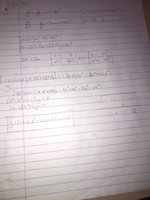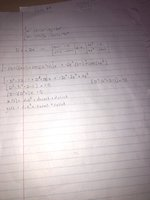# Solving a system of non-homogeneous differential equations....

#### Vraellie

##### New member
So, it's my first time posting here, and I would be incredibly grateful for an extra pair of eyes to tell me if I'm understanding this correctly. The problem is as follows:After putting my equations into standard form and using the modified kramer's method to find both solution's for x and y, I'm pretty unnerved by the answer, because it is the same answer, and although these equations are non-homogeneous, there is no particular solution. Usually, we have to express one of the constants, either c or d, in

#### HallsofIvy

##### Elite Member
The set of equations is
$$\displaystyle \frac{dx}{dt}- \frac{dy}{dt}- x= 2e^t$$ and
$$\displaystyle \frac{d^2x}{dt^2}- \frac{dy}{dt}+ 3x- y= 4e^t$$.

The first thing I would do is solve the first equation for $$\displaystyle \frac{dy}{dt}$$:
$$\displaystyle \frac{dy}{dt}= \frac{dx}{dt}- x- 2e^t$$. Replace $$\displaystyle \frac{dy}{dt}$$ by that in the second equation: $$\displaystyle \frac{d^2x}{dt^2}- \left(\frac{dx}{dt}- x- 2e^t\right)+ 3x- y= 4e^t$$ or $$\displaystyle \frac{d^2x}{dt^2}-\frac{dx}{dt}+ 4x- y= 2e^t$$.

Now, differentiate that equation again: $$\displaystyle \frac{d^3x}{dt^3}- \frac{d^2x}{dt^2}+ 4\frac{dx}{dt}- \frac{dy}{dt}= 2e^t$$. And, finally, replace that "dy/dt" with $$\displaystyle \frac{dx}{dt}- x- 2e^t$$ again: $$\displaystyle \frac{d^3x}{dt^3}- \frac{d^2x}{dt^2}+ 4\frac{dx}{dx}- \frac{dx}{dt}+ x+ 2e^t= 2e^t$$.

$$\displaystyle \frac{d^3x}{dt^3}- \frac{d^2x}{dt^2}+ 3\frac{dx}{dt}+ x= 0$$.

That is a linear homogeneous equation with constant coefficients. It has characteristic equation $$\displaystyle r^3- r^2+ 3r+ 1= 0$$. I will leave you to finish it. (Check my arithmetic!)